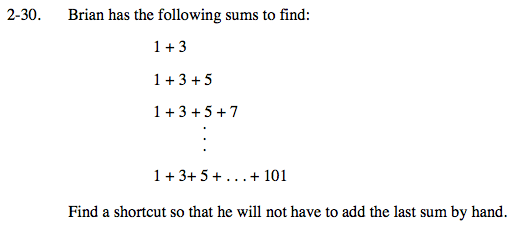### Home > PC > Chapter 2 > Lesson 2.1.2 > Problem2-30

2-30.1 + 3 = 4 or 22 (How many terms are in the sum?)

1 + 3 + 5 = 9 or 32 (How many terms are in the sum?)

1 + 3 + 5 + 7 = 16 or 42 (How many terms are in the sum?)

(How many terms are in the final sum?)